New Cartesian Physics and Universe Pressure | OMICS International
Global Journal of Technology and Optimization
All submissions of the EM system will be redirected to Online Manuscript Submission System. Authors are requested to submit articles directly to Online Manuscript Submission System of respective journal.

# New Cartesian Physics and Universe Pressure

Dizhechko Boris Semyonowich*

City of Sterlitamak, Bashkortostan, Russia

Corresponding Author:
Dizhechko Boris Semyonowich
City of Sterlitamak
Bashkortostan, Russia
Tel: 7 4997041341
E-mail: [email protected]

Received Date: July 12, 2016; Accepted Date: August 18, 2016; Published Date: August 22, 2019

Citation: Semyonowich DB (2016) New Cartesian Physics and Universe Pressure. Global J Technol Optim 7:201. doi: 10.4172/2229-8711.1000201

Copyright: © 2016 Semyonowich DB. This is an open-access article distributed under the terms of the Creative Commons Attribution License, which permits unrestricted use, distribution, and reproduction in any medium, provided the original author and source are credited.

Visit for more related articles at Global Journal of Technology and Optimization

#### Abstract

Each of us for the first time after seeing the formula mass-energy equivalence E = m0c2 came to the admiration and amazement of how mass any of the body becomes energy. The answer to this question is absent both in the theory of relativity and in quantum mechanics. Once my colleague Edgars Alksnis noticed that New Cartesian physics will have success if explain the formula the mass-energy equivalence. It’s easy to make - thought I, at my was already formulated the law of constancy of flow of forces through a closed surface, which was a generalization of the Gauss law, according to which each movement begins as a result of deviations from this permanence.

#### Keywords

Mass-energy; Gauss law; Heisenberg uncertainty

#### Introduction

Each of us for the first time after seeing the formula mass-energy equivalence E = m0c2 came to the admiration and amazement of how mass any of the body becomes energy. The answer to this question is absent both in the theory of relativity and in quantum mechanics. Once my colleague Edgars Alksnis noticed that New Cartesian physics will have success if explain the formula the mass-energy equivalence. It’s easy to make - thought I, at my was already formulated the law of constancy of flow of forces through a closed surface, which was a generalization of the Gauss law, according to which each movement begins as a result of deviations from this permanence. I took the formula mass-energy equivalence E = m0c2 and began from identical conversion as a result of which showed that energy within particles is constrained by pressure of the universe in the same way as the pressure of air creates the conditions for the formation of different rotational movements (tornadoes, cyclone, etc.), i.e. the recognition of the concept of equivalence of mass and energy requires the recognition of the existence of pressure the universe.

Can be written: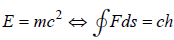It is clear that in corpuscle there are several such rotational movements, with the speed of light. Centrifugal forces’ arising while spinning space presses at the external side and meet them the pressure of the universe. It is the answer is found in the new Cartesian physics, based on the principle of identity space and matter. This principle allows treating space as matter, i.e. believing that it moves according to the laws of mechanics. But, tell you, corporal particles possess hardness, whether the space possesses it? Yes, argues new Cartesian physics, must otherwise look at Heisenberg’s uncertainty principle and turn it into the certainty principle points of space.

Cartesian identity «space ≡ matter» easily transforms the Heisenberg uncertainty principle in the certainty principle points of moving space-matter. Take the known attitude of the Heisenberg uncertainty between the position x1 and momentum p1 of a particle in space: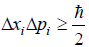Where «ħ» is Planck’s constant (h) divided on 2π.

Note, that here on the right and at the left we have the moment of a momentum and therefore defines radius of rotation, and is a coordinate of the center of rotation of space-matter. The principle of uncertainty Heisenberg between coordinate and momentum of a particle in space can be written down then so,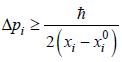From this formula we see, that infinitely big momentum is necessary for approach of current coordinate to coordinate of the center of rotation of space-matter,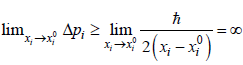This inequality shows that at reduction of the interval containing a point with coordinate’s xi0, there is an increase in an increment of the momentum necessary for localization of this point of space-matter from its other points. In the infinitesimal interval containing this point, this increment becomes infinite greater. The formula transforms a principle of uncertainty Heisenberg to a principle of definiteness of each point of space-matter with coordinate’s xi0. The principle of definiteness shows that localization of area containing a point of space-matter with coordinate’s xi0 occurs by influence of the momentum exceeding or equal specified size. This principle is a principle of physical irrationality of points of space-matter which specifies their continuity and infinite divisibility.

Thus, the space in their points has infinitely great hardness, which decreases with increasing their number, i.e. with the extension field containing them. This includes not only the space, but also to the corpuscular, which represent the rotating parts of the space.

Since the opening of R. Einstein law of equivalence of mass and energy were of the view that the forces holding atoms mass is inside their nuclei. Physicists of the different countries till now make expensive experiments on colliding elementary particles to push out from them particles which ostensibly form mass.

New Cartesian physics finds it problem is incorrect. From the Heisenberg inequality and speed limit any movement at the speed of light, you can see, that there is no mass of quantum and cannot find such particle which would reflect mass of any other particles. Is quantum the product of the masses on a characteristic particle size mr = ħ / c , which suggests that the smaller the radius of curvature of the movement of space, the more it has mass? What is the mass? New Cartesian physics defined mass through of flow the acceleration vectors moving space in orbit, the radius of which is equal to the Compton wavelength. The Compton wavelength of a particle is equivalent to the wavelength of a photon whose energy is the same as the rest-mass energy of the particle.

In corpuscle the flow of forces of the universe is balanced by centrifugal force flow internal rotation of space, reaching the speed of light at a distance of Compton wavelength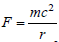From this we get equality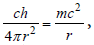rewrite this expression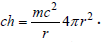Here the left stands the flow force of the universe, and on the right flow of centrifugal force. Make a mass m for the integral symbol, we obtain: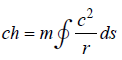Hence the mass of the particles is equal to the flow of forces of the universe, divided on the flow of centrifugal acceleration: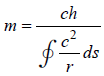This is Newton’s second law for corpuscles.

#### Conclusion

Thus, the pressure of the universe is the cause of all the movements taking place in the real world. The greatest manifests itself within the corpuscle where under his influence shaped portion of energy that defines its mass. When this the surface integral, taken on a closed surface, does not depend on the distance to the Center, so and the pressure of the universe we can talk as attributes. Hence we receive the laws of conservation of mass and a constant pressure of the universe.

Select your language of interest to view the total content in your interested language

### Article Usage

• Total views: 8468
• [From(publication date):
December-2016 - Nov 21, 2019]
• Breakdown by view type
• HTML page views : 8451Can't read the image? click here to refresh